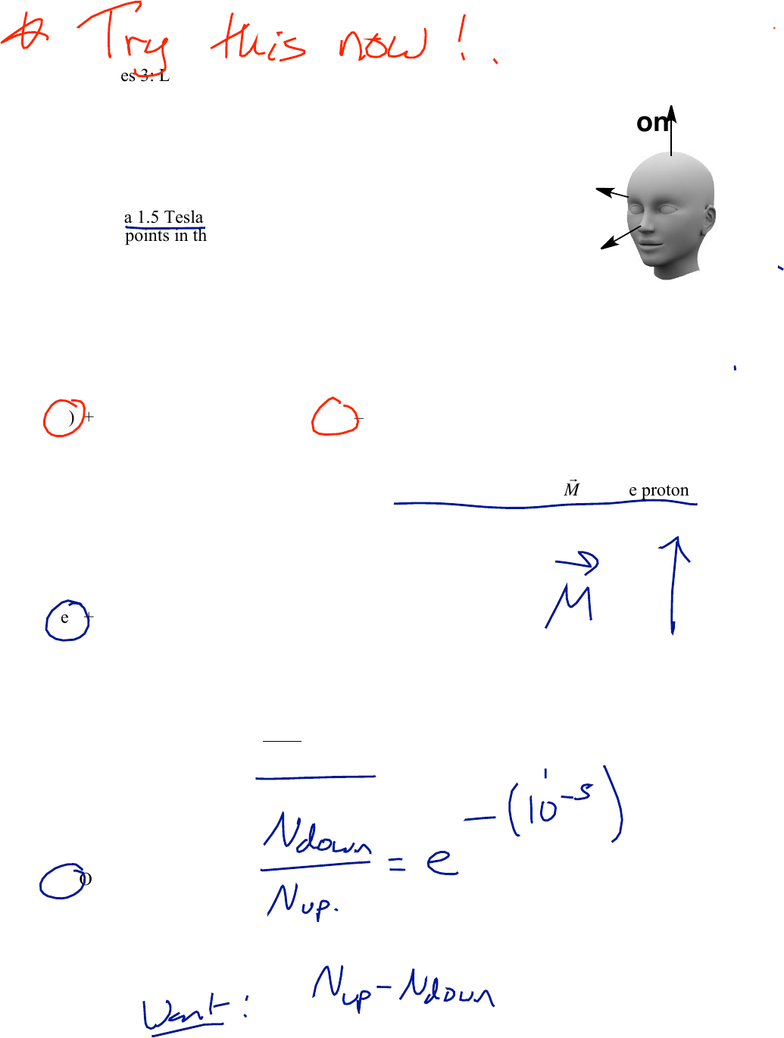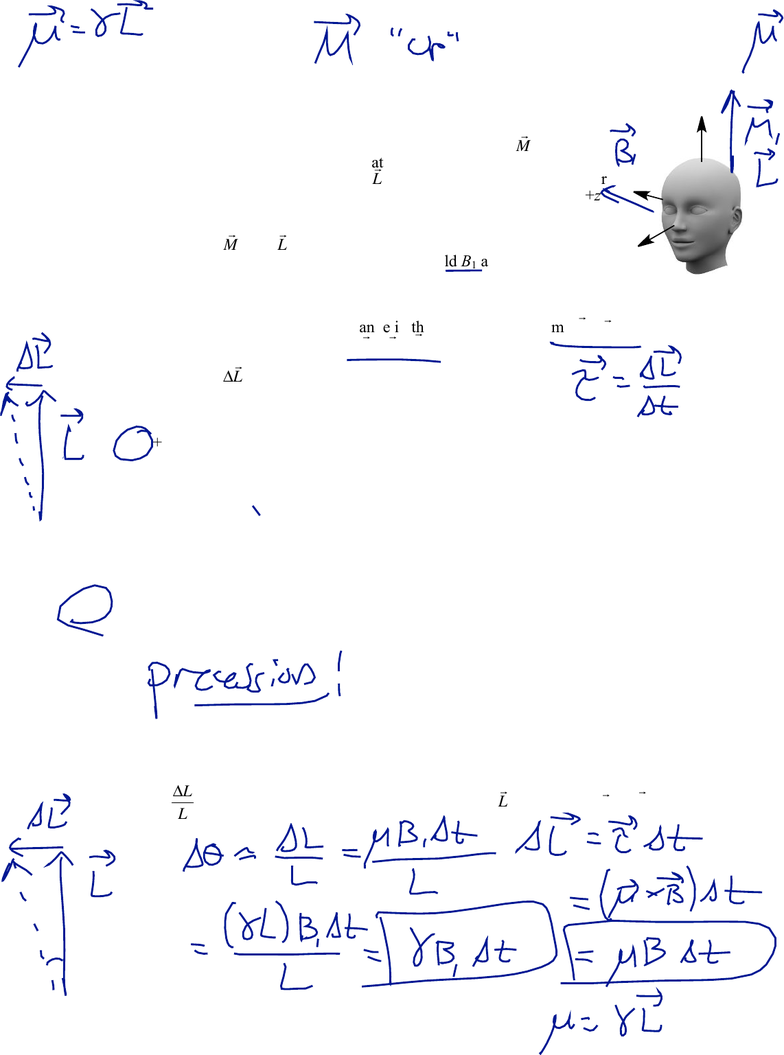Study Guides (400,000)
US (230,000)
Harvard (700)
(20)
Final

# Physical Sciences 3 Lecture Notes - Lecture 14: Magnetic Resonance Imaging, Boltzmann Distribution, ParamagnetismExam

Department
Physical Sciences
Course Code
Physical Sciences 3
Professor
Roxanne Guenette
Study Guide
Final

This preview shows pages 1-3. to view the full 11 pages of the document.
Physical Sciences 3: Lecture 5d
March 26, 2019
1
Magnetic Resonance Imaging
At thermal equilibrium, there is a distribution of protons among the two possible
stationary states according to the Boltzmann distribution. As a result, there will be a net
magnetization given by the sum of all of the individual magnetic dipoles. This net
magnetization will point along the z-axis (the direction of the original applied field).
If you apply an oscillating magnetic field at the Larmor frequency, you can rotate the
magnetization vector away from its equilibrium state. The magnetization will relax back
to equilibrium through two processes known as T1 and T2. The different relaxation
times will be different for different kinds of tissue, so an MRI image can detect the
presence of a tumor or other pathology.
By applying various field gradients we can select a particular slice of the sample to be
excited at its Larmor frequency. Then, field gradients applied during data collection allow
us to “read out” the location of each tissue based on their different Larmor frequencies.
Different kinds of tissues have different relaxation times, so radiologists can distinguish
between fat and bone, muscle and tendon, white matter and gray matter, and identify
tumors and other pathologies. In addition, we can use contrast agents that contain
paramagnetic ions to add additional contrast to particular tissues or regions such as the
circulatory system.
Learning objectives: After this lecture, you will be able to…
1. Describe how you can “nudge” the magnetization vector of protons inside a patient by
applying a magnetic field perpendicular to the primary field B0.
2. Explain why the “nudged” magnetization vector will precess at the Larmor frequency.
3. Describe how you can rotate the magnetization vector to any arbitrary angle by applying
an oscillating magnetic field perpendicular to the primary field.
4. Explain why the oscillating field must have the same frequency as the Larmor frequency
in order to effectively rotate the magnetization.
5. Calculate the duration of the oscillating pulse required to rotate the magnetization vector
through a specific total angle.
6. Describe the different relaxation processes and their associated time constants: T1, T2, and
T2*. Calculate the components of the magnetization based on these time constants.
7. Explain how field gradients are used to selectively rotate the “spins” within a certain slice
of tissue. Calculate the gradient and Larmor frequency required to image a specific slice.
8. Explain how field gradients are used to selectively “listen” to the precession and
relaxation of spins along a single line within the patient, and how tomography can be
used to determine the values of T1 and T2 at a single point.
9. Explain how the values of T1 and T2 can be used to identify different tissues, and how
paramagnetic contrast agents can be used to highlight particular areas of clinical interest.

Only pages 1-3 are available for preview. Some parts have been intentionally blurred.Physical Sciences 3: Lecture 5d
March 26, 2019
2
L5d: Am I getting it? #1 Equilibrium Magnetization
Your head is inside an MRI machine, with the z axis running straight
up, the x axis through your right ear, and the y-axis through your nose.
There is a 1.5 Tesla magnetic field B0 directed along the z-axis (i.e.
the field points in the positive z-direction)
1. At equilibrium, which of the following directions would be allowed as
a stationary state of the proton spins (magnetic dipoles)? Choose all
that apply.
a) +x direction b) x direction
c) +y direction d) y direction
e) +z direction f) z direction
2. At equilibrium, what is the direction of the net magnetization vector
M
for the protons in
a) +x direction b) x direction
c) +y direction d) y direction
e) +z direction f) z direction
3. What is the approximate fraction of excess “spin up” protons in your head?
(Note that in this situation,
γ
!B
0
k
B
T10
–5
)
a) One in 10
b) One in 103
c) One in 105
d) One in 107
z
x
y

Only pages 1-3 are available for preview. Some parts have been intentionally blurred.Physical Sciences 3: Lecture 5d
March 26, 2019
3
Activity 1: Nudging the Magnetization Vector
We’re going to be focusing on the magnetization vector
M
for the rest
of this class. Note that the magnetization vector will also point in the
direction of the angular momentum
L
. We will always assume that our
axes are defined such that the primary MRI field B0 points along the +z
axis.
1. Suppose that
M
and
L
initially point along the +z axis (as expected at
equilibrium). If you apply a small magnetic field B1 along the +x axis,
you will “nudge” the magnetization vector a little bit. What is the
direction of this “nudge”?
Hint: Think of the “nudge” as a change in the angular momentum
Δ
L=
τ
Δt
. Recall that
the torque on a magnetic dipole is
.
The “nudge”
Δ
L
will be in the (circle one):
a) +x direction b) x direction
c) +y direction d) y direction
e) +z direction f) z direction
2. Now that the angular momentum has been “nudged,” it no longer points along the z axis!
That means it is no longer in a stationary state. What will happen to it now?
Bonus! Find an expression for the approximate angle Δ
θ
of the “nudge,” if the field B1 is applied
for a short time Δt. Since the nudge is small, then the change in angle is approximately:
Δ
θ
=ΔL
L
, where ΔL is the magnitude of the change in
L
. Recall that
µ
=
γ
L
.
z
x
y